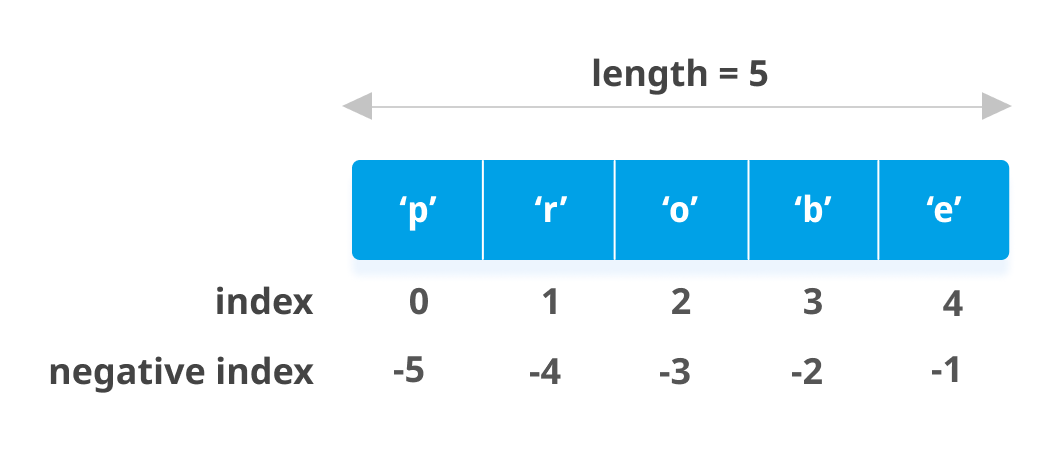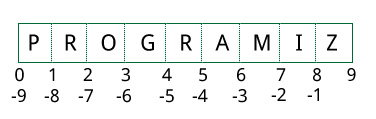# Python List

In this article, we'll learn everything about Python lists; how they are created, slicing of a list, adding or removing elements from them and so on.

Python offers a range of compound datatypes often referred to as sequences. List is one of the most frequently used and very versatile datatype used in Python.

## How to create a list?

In Python programming, a list is created by placing all the items (elements) inside a square bracket [ ], separated by commas.

It can have any number of items and they may be of different types (integer, float, string etc.).

``````# empty list
my_list = []

# list of integers
my_list = [1, 2, 3]

# list with mixed datatypes
my_list = [1, "Hello", 3.4]
``````

Also, a list can even have another list as an item. This is called nested list.

```# nested list
my_list = ["mouse", [8, 4, 6], ['a']]
```

## How to access elements from a list?

There are various ways in which we can access the elements of a list.

### List Index

We can use the index operator [] to access an item in a list. Index starts from 0. So, a list having 5 elements will have index from 0 to 4.

Trying to access an element other that this will raise an `IndexError`. The index must be an integer. We can't use float or other types, this will result into `TypeError`.

Nested list are accessed using nested indexing.

``````my_list = ['p','r','o','b','e']
# Output: p
print(my_list)

# Output: o
print(my_list)

# Output: e
print(my_list)

# Error! Only integer can be used for indexing
# my_list[4.0]

# Nested List
n_list = ["Happy", [2,0,1,5]]

# Nested indexing

# Output: a
print(n_list)

# Output: 5
print(n_list)
``````

### Negative indexing

Python allows negative indexing for its sequences. The index of -1 refers to the last item, -2 to the second last item and so on.

``````my_list = ['p','r','o','b','e']

# Output: e
print(my_list[-1])

# Output: p
print(my_list[-5])
``````## How to slice lists in Python?

We can access a range of items in a list by using the slicing operator (colon).

``````my_list = ['p','r','o','g','r','a','m','i','z']
# elements 3rd to 5th
print(my_list[2:5])

# elements beginning to 4th
print(my_list[:-5])

# elements 6th to end
print(my_list[5:])

# elements beginning to end
print(my_list[:])
``````

Slicing can be best visualized by considering the index to be between the elements as shown below. So if we want to access a range, we need two indices that will slice that portion from the list.## How to change or add elements to a list?

List are mutable, meaning, their elements can be changed unlike string or tuple.

We can use assignment operator (=) to change an item or a range of items.

``````# mistake values
odd = [2, 4, 6, 8]

# change the 1st item
odd = 1

# Output: [1, 4, 6, 8]
print(odd)

# change 2nd to 4th items
odd[1:4] = [3, 5, 7]

# Output: [1, 3, 5, 7]
print(odd)
``````

We can add one item to a list using `append()` method or add several items using `extend()` method.

``````odd = [1, 3, 5]

odd.append(7)

# Output: [1, 3, 5, 7]
print(odd)

odd.extend([9, 11, 13])

# Output: [1, 3, 5, 7, 9, 11, 13]
print(odd)
``````

We can also use + operator to combine two lists. This is also called concatenation.

The * operator repeats a list for the given number of times.

``````odd = [1, 3, 5]

# Output: [1, 3, 5, 9, 7, 5]
print(odd + [9, 7, 5])

#Output: ["re", "re", "re"]
print(["re"] * 3)
``````

Furthermore, we can insert one item at a desired location by using the method `insert()` or insert multiple items by squeezing it into an empty slice of a list.

``````odd = [1, 9]
odd.insert(1,3)

# Output: [1, 3, 9]
print(odd)

odd[2:2] = [5, 7]

# Output: [1, 3, 5, 7, 9]
print(odd)
``````

## How to delete or remove elements from a list?

We can delete one or more items from a list using the keyword `del`. It can even delete the list entirely.

``````my_list = ['p','r','o','b','l','e','m']

# delete one item
del my_list

# Output: ['p', 'r', 'b', 'l', 'e', 'm']
print(my_list)

# delete multiple items
del my_list[1:5]

# Output: ['p', 'm']
print(my_list)

# delete entire list
del my_list

# Error: List not defined
print(my_list)
``````

We can use `remove()` method to remove the given item or `pop()` method to remove an item at the given index.

The `pop()` method removes and returns the last item if index is not provided. This helps us implement lists as stacks (first in, last out data structure).

We can also use the `clear()` method to empty a list.

``````my_list = ['p','r','o','b','l','e','m']
my_list.remove('p')

# Output: ['r', 'o', 'b', 'l', 'e', 'm']
print(my_list)

# Output: 'o'
print(my_list.pop(1))

# Output: ['r', 'b', 'l', 'e', 'm']
print(my_list)

# Output: 'm'
print(my_list.pop())

# Output: ['r', 'b', 'l', 'e']
print(my_list)

my_list.clear()

# Output: []
print(my_list)
``````

Finally, we can also delete items in a list by assigning an empty list to a slice of elements.

``````>>> my_list = ['p','r','o','b','l','e','m']
>>> my_list[2:3] = []
>>> my_list
['p', 'r', 'b', 'l', 'e', 'm']
>>> my_list[2:5] = []
>>> my_list
['p', 'r', 'm']
``````

## Python List Methods

Methods that are available with list object in Python programming are tabulated below.

They are accessed as `list.method()`. Some of the methods have already been used above.

Some examples of Python list methods:

``````my_list = [3, 8, 1, 6, 0, 8, 4]

# Output: 1
print(my_list.index(8))

# Output: 2
print(my_list.count(8))

my_list.sort()

# Output: [0, 1, 3, 4, 6, 8, 8]
print(my_list)

my_list.reverse()

# Output: [8, 8, 6, 4, 3, 1, 0]
print(my_list)
``````

## List Comprehension: Elegant way to create new List

List comprehension is an elegant and concise way to create a new list from an existing list in Python.

List comprehension consists of an expression followed by for statement inside square brackets.

Here is an example to make a list with each item being increasing power of 2.

``````pow2 = [2 ** x for x in range(10)]

# Output: [1, 2, 4, 8, 16, 32, 64, 128, 256, 512]
print(pow2)
``````

This code is equivalent to

``````pow2 = []
for x in range(10):
pow2.append(2 ** x)
``````

A list comprehension can optionally contain more `for` or if statements. An optional `if` statement can filter out items for the new list. Here are some examples.

``````>>> pow2 = [2 ** x for x in range(10) if x > 5]
>>> pow2
[64, 128, 256, 512]
>>> odd = [x for x in range(20) if x % 2 == 1]
>>> odd
[1, 3, 5, 7, 9, 11, 13, 15, 17, 19]
>>> [x+y for x in ['Python ','C '] for y in ['Language','Programming']]
['Python Language', 'Python Programming', 'C Language', 'C Programming']
``````

## Other List Operations in Python

### List Membership Test

We can test if an item exists in a list or not, using the keyword `in`.

``````my_list = ['p','r','o','b','l','e','m']

# Output: True
print('p' in my_list)

# Output: False
print('a' in my_list)

# Output: True
print('c' not in my_list)
``````

### Iterating Through a List

Using a `for` loop we can iterate though each item in a list.

``````for fruit in ['apple','banana','mango']:
print("I like",fruit)```
```